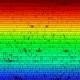Basic feature comparisons (e.g. amount of L3 cache):```png( filename="plot.png", width=640, height=440, bg="#070707" );
par(bg="#070707", fg="white", col.axis="white", col.main="white", col.lab="white", mar=c(3,4,2,2) + 0.1);
plot(xeon\$Model, xeon\$L3.Cache, ylab="L3 Cache", border=brewer.pal(8, "Dark2"), cex.axis=1.3, cex.lab=1.5);
dev.off();
```

Two-factor comparisons (e.g. with and without turbo boost):```png( filename="plot.png", width=640, height=440, bg="#070707" );
par(bg="#070707", fg="white", col.axis="white", col.main="white", col.lab="white", mar=c(3,4,2,2) + 0.1);
plot(xeon\$Model, xeon\$Frequency, ylab="Processor Frequency", ylim=c(1.8,2.8), border=brewer.pal(8, "Dark2"), cex.axis=1.3, cex.lab=1.5)
points(xeon\$Model, xeon\$Frequency..Turbo., col=brewer.pal(8, "Dark2"), pch=19)
dev.off();
```

Required packages: RColorBrewer

Intel Xeon 7500-series model lineup

Tags:

### One Response to “R: Plots for Intel Xeon 7500 Comparison”

1.`points(xeon\$Model, xeon\$Frequency..Turbo., col=rev(brewer.pal(8, "Dark2")), pch=19)`